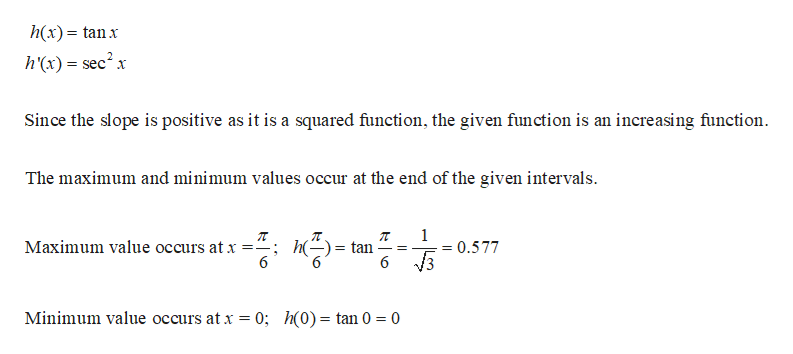# Let h(t) = tan t.Find the maximum and minimum values of h on the interval I = [0, π/6]. Then use the Comparison Property to find upper and lower bounds for the area of the region between the graph of h and the x axis on the interval I.

Question
716 views

Let h(t) = tan t.

Find the maximum and minimum values of h on the interval I = [0, π/6]. Then use the Comparison Property to find upper and lower bounds for the area of the region between the graph of h and the x axis on the interval I.

check_circle

Step 1

Given funct...help_outlineImage Transcriptioncloseh(x) tanx h'(x) = sec2x Since the slope is positive as it is a squared function, the given function is an increasing function The maximum and minimum values occur at the end of the given intervals 1 Maximum value occurs at x 6 = tan 6 0.577 V3 Minimum value occurs at x = 0; h(0) = tan 0 = 0 fullscreen

### Want to see the full answer?

See Solution

#### Want to see this answer and more?

Solutions are written by subject experts who are available 24/7. Questions are typically answered within 1 hour.*

See Solution
*Response times may vary by subject and question.
Tagged in

### Functions